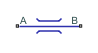# Flow Resistance (IL)

General resistance in an isothermal liquid branch

Since R2020a

•Libraries:
Simscape / Foundation Library / Isothermal Liquid / Elements

## Description

The Flow Resistance (IL) block models a general pressure drop in an isothermal-liquid network branch. The pressure drop is proportional to the square of the mass flow rate. The constant of proportionality is determined from a nominal operating condition specified in the block dialog box.

Use this block when the only data available for a component is the typical pressure drop and flow rate. This block is useful for representing complex components, where it is difficult to determine theoretical pressure loss from the geometry.

The volume of fluid inside the flow resistance is assumed to be negligible. The mass flow rate in through one port must then exactly equal the mass flow rate out through the other port:

`${\stackrel{˙}{m}}_{\text{A}}+{\stackrel{˙}{m}}_{\text{B}}=0,$`

where ${\stackrel{˙}{m}}_{\text{A}}$ and ${\stackrel{˙}{m}}_{\text{B}}$ are defined as the mass flow rates into the component through ports A and B, respectively.

The pressure drop is assumed proportional to the square of the mass flow rate. The square of the mass flow rate is linearized in a small laminar flow region near zero flow, resulting in

`${p}_{A}-{p}_{B}=K{\stackrel{˙}{m}}_{A}\sqrt{{\stackrel{˙}{m}}_{\text{A}}^{2}+{\stackrel{˙}{m}}_{\text{lam}}^{2}},$`

where:

• pA and pB are pressures at ports A and B, respectively.

• ${\stackrel{˙}{m}}_{lam}$ is the mass flow rate threshold for laminar transition.

K is the proportionality constant,

`$K=\frac{\Delta {p}_{nom}}{{\stackrel{˙}{m}}_{nom}^{2}},$`

where Δpnom is the Nominal pressure drop parameter value and $\stackrel{˙}{m}$nom is the Nominal mass flow rate parameter value.

## Ports

### Conserving

expand all

Isothermal liquid conserving port associated with the inlet or outlet of the flow resistance. This block has no intrinsic directionality.

Isothermal liquid conserving port associated with the inlet or outlet of the flow resistance. This block has no intrinsic directionality.

## Parameters

expand all

Pressure drop from inlet to outlet at a known operating condition. The block uses the nominal parameters to calculate the constant of proportionality between the pressure drop and the mass flow rate.

Mass flow rate through the component at a known operating condition. The block uses the nominal parameters to calculate the constant of proportionality between the pressure drop and the mass flow rate.

Ratio of the threshold mass flow rate to the nominal mass flow rate. The block uses this parameter to calculate the threshold mass flow rate—and ultimately to set the limits of linearization for the pressure drop.

## Version History

Introduced in R2020a